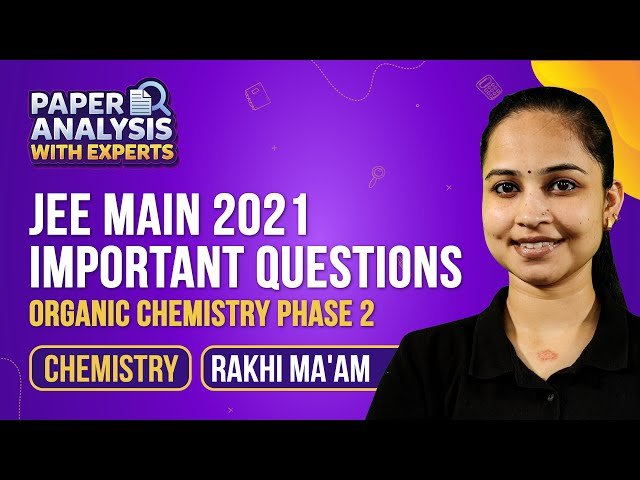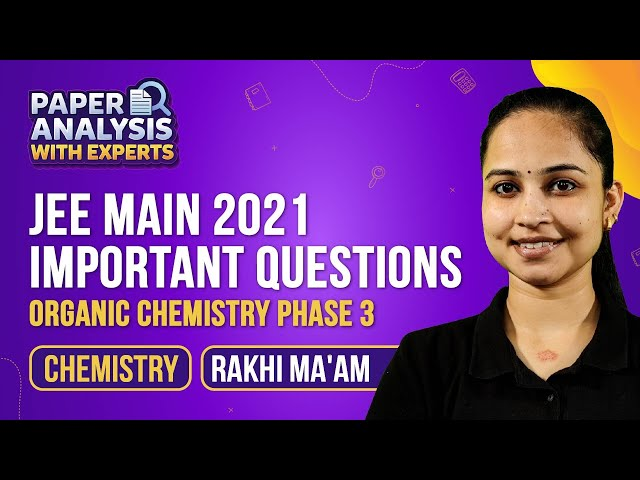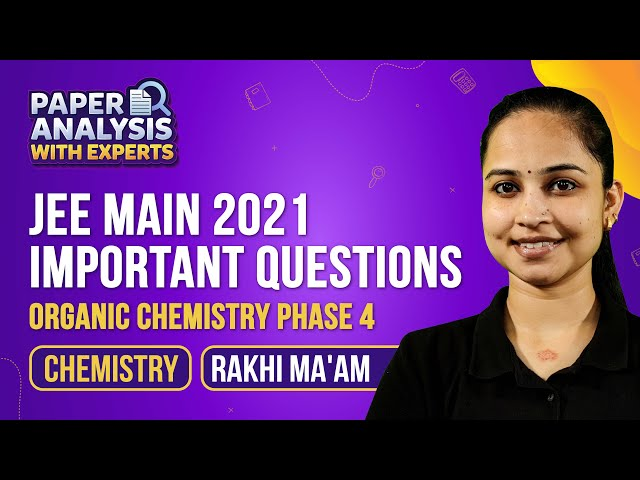# JEE Main Organic Chemistry Previous Year Questions with Solutions

General Organic Chemistry Previous Year Questions with Solutions are given here. BYJU’S provides accurate solutions prepared by our subject matter experts. General Organic Chemistry covers the fundamental concepts in the vast field of organic chemistry. A strong understanding of the concepts that come under GOC plays a vital role in the study of relatively advanced topics (such as the mechanisms of named reactions).

The chapter contains important topics such as inductive effect, electrophiles and nucleophiles, isomerism, the resonance effect, etc. This chapter is one of the highest weightage chapters. Students can expect 2 or 3 questions from this chapter. The questions in this article help students to understand the difficulty level of questions in the upcoming JEE Main and JEE Advanced exams. Students can easily download the solutions in PDF format.

## JEE Main General Organic Chemistry Previous Year Questions With Solutions

1. The most basic compound among the following is:-

(1) Acetanilide

(2) Benzylamine

(3) p-Nitro aniline

(4) Aniline

Solution:

Basicity is inversely proportional to resonance of lone pair electrons. Benzylamine is more basic. The electron pairs do not involve in resonance in benzylamine. In other amines, there is delocalization of lone pair of electron on N atom on the ring. In acetanilide, the delocalization of lone pair of electrons on N atom is due to adjacent CO group.

Hence option (2) is the answer.

2. Considering the basic strength of amines in aqueous solution, which one has

the smallest pKb value?

(1) (CH3)3N

(2) C6H5NH2

(3) (CH3)2NH

(4) CH3NH2

Solution:

Because of resonance, aryalamines are less basic than alkyl amines. In aryalamines, the lone pair of electrons on N is partly shared with the ring and is less available for sharing with a proton. In alkylamines, the electron releasing alkyl group increases the electron density on nitrogen atom. Hence increases the protonation ability of amines. So, more the number of alkyl groups, higher will be basicity of amine. Because of steric effect, a slight discrepancy occurs in case of trimethyl amine. So (CH3)2NH has the smallest pKb value.

Hence option (3) is the answer.

3. Among the following the molecule with the lowest dipole moment is:-

(1) CHCl3

(2) CH2Cl2

(3) CCl4

(4) CH3Cl

Solution:

Dipole moment of CCl4 is 0.

The order of dipole moment is CCl4 < CH3Cl < CH2Cl2 < CHCl3.

Hence option (3) is the answer.

4. Which one of the following compounds will not be soluble in sodium bicarbonate ?

(1) Benzene sulphonic acid

(2) Benzoic acid

(3) o-Nitrophenol

(4) 2, 4, 6 – Trinitrophenol

Solution:

Benzene sulphonic acid and Benzoic acid are stronger acids and they react with sodium bicarbonate. o-Nitrophenol is a very weak acid and it does not react with sodium bicarbonate. o-Nitrophenol will not be soluble in sodium bicarbonate. 2, 3, 6 – Trinitrophenol is higher in acidity and it reacts with sodium bicarbonate.

Hence option (3) is the answer.

5. The compound formed in the positive test for nitrogen with the Lassaigne solution of an organic compound is

(1) Fe4[Fe(CN)6]3

(2) Na4[Fe(CN)5NOS]

(3) Fe(CN)3

(4) Na3[Fe(CN)6]

Solution:

The compound formed in the positive test for nitrogen with the Lassaigne solution of an organic compound is prussian blue coloured complex compound ferric ferrocyanide.

Hence option (1) is the answer.

6. The general formula CnH2n02 could be for open chain

(1) carboxylic acids

(2) diols

(3) dialdehydes

(4) diketones

Solution:

The general formula CnH2n02 could be for open chain carboxylic acid or ester.

Hence option (1) is the answer.

7.Among the following oxoacids, the correct decreasing order of acid strength is :

(1) HClO4 > HClO3 > HClO2 > HOCl

(2) HClO2 > HClO4 > HClO3 > HOCl

(3) HOCl > HClO2 > HClO3 > HClO4

(4) HClO4 > HOCl > HClO2 > HClO3

Solution:

Acidic strength is directly proportional to the oxidation number. Increasing acid strength is because of an increase in the oxidation state of the central atom.

Hence option (1) is the answer.

8. Ortho-Nitrophenol is less soluble in water than p– and m– Nitrophenols because:

(1) Melting point of o–Nitrophenol is lower than those of m– and p– isomers

(2) o–Nitrophenol is more volatile in steam than those of m– and p– isomers

(3) o–Nitrophenol shows Intramolecular H–bonding

(4) o–Nitrophenol shows Intermolecular H–bonding

Solution:

Intramolecular H-bonding is present in o-nitrophenol. So solubility in water is decreased.

Hence option (3) is the answer.

9. The order of basicity of amines in gaseous state is :

(1) 30 > 20 > NH3 > 10

(2) 10 > 20 > 30 > NH3

(3) NH3> 10 > 20 > 30

(4) 30 > 20 > 10 > NH3

Solution:

The basicity is proportional to +I effect. The presence of electron-donating group increases the basicity of amines. The presence of withdrawing group decreases the basicity of amines.

Hence option (4) is the answer.

10. Which one of the following conformation of cyclohexane is chiral ?

(1) Twist boat

(2) Rigid

(3) Chair

(4) Boat

Solution:

Twist boat is chiral because it does not have a plane of symmetry.

Hence option (1) is the answer.

11. Increasing order of stability among the three main conformations (i.e. Eclipse, Anti, Gauche) of 2-fluoroethanol is

(1)Eclipse, Gauche, Anti

(2)Gauche, Eclipse, Anti

(3)Eclipse, Anti, Gauche

(4)Anti, Gauche, Eclipse

Solution:

Eclipse is least stable and Gauche is most stable.

Hence option (3) is the answer.

12. The conjugate base of hydrazoic acid is :-

(1) NH3

(2) N3

(3) N2

(4) N-3

Solution:

Hydraulic acid is HN3.

HN3 → H+ + N3

Hence option (2) is the answer.

13. The correct order of increasing basicity of the given conjugate base (R =CH3) is:

(1) RCOO < HC ≡ C < NH2 < R

(2) RCOO < HC ≡ C < R< NH2

(3) R < HC ≡ C < RCOO < NH2

(4) RCOO < NH2 < HC ≡ C< R

Solution:

The basic strength is inversely proportional to the stability of conjugate base. In basicity, if the availability of the electrons is more, then more readily they can be donated to form a new bond to the proton and, and hence the stronger base. If bronsted acid is a strong acid then its conjugate base is a weak base .

The correct order of increasing basic strength is RCOO < HC ≡ C < NH2 < R

Hence option (1) is the answer.

14. Which types of isomerism is shown by 2,3-dichlorobutane?

(1) Diastereo

(2) Optical

(3) Geometric

(4) Structural

Solution:

2,3- dichlorobutane shows optical isomerism.

Hence option (2) is the answer.

15. Due to the presence of an unpaired electron, free radicals are

(1)Chemically reactive

(2) Chemically inactive

(3) Anions

(4) Cations

Solution:

Due to the presence of an unpaired electron, the free radicals are chemically active.

Hence option (1) is the answer.

16. Which one the following does not have sp2 hybridized carbon?

(1) Acetone

(2) Acetamide

(3) Acetonitrile

(4) Acetic acid

Solution:

Acetonitrile is having only sp3 and sp hybridized carbon atoms.

Hence option (3) is the answer.

General Organic Chemistry GOC for JEE

Organic Chemistry

## Organic Chemistry JEE Main Important Questions – Phase 1## Organic Chemistry JEE Main Important Questions – Phase 2## Organic Chemistry JEE Main Important Questions – Phase 3## Organic Chemistry JEE Main Important Questions – Phase 4Test Your Knowledge On Organic Chemistry Previous Year Questions With Solutions!